# Class 9 RD Sharma Solutions – Chapter 7 Introduction to Euclid’s Geometry- Exercise 7.1

• Last Updated : 15 Dec, 2020

### (vii) Half-line

Solution:

(i) A line segment is a one-dimensional line connecting two points. It is the shortest distance that is incident on both the points.

Attention reader! All those who say programming isn't for kids, just haven't met the right mentors yet. Join the  Demo Class for First Step to Coding Coursespecifically designed for students of class 8 to 12.

The students will get to learn more about the world of programming in these free classes which will definitely help them in making a wise career choice in the future.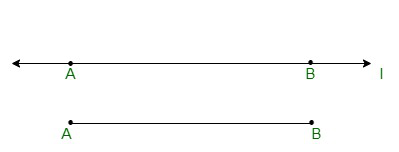(ii) Two or more points incident on the same line are called collinear points.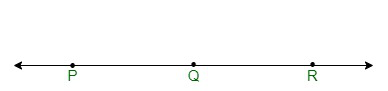(iii) Parallel lines run opposite each other and do not intersect each other at any point of time.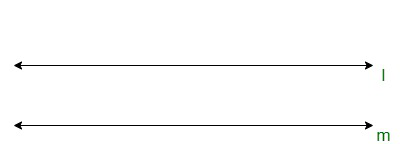(iv) Intersecting line segments are a pair of lines meeting each other at a common point, which is also called a point of intersection. Both the lines are incident on this point.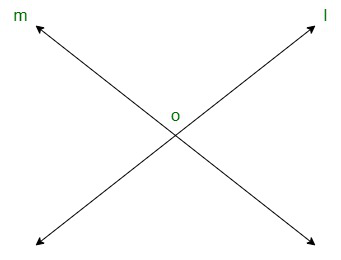(v) Two or multiple line segments that are incident on a common point, are called concurrent lines.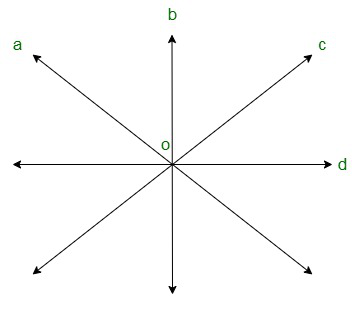(vi) A ray is a line segment that is fixed on one end and indefinitely extending on the other.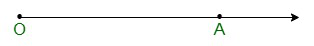(vii) Let us assume A, B, C be the points on a line, such that A lies mid-way between B and C. If we delete the point A in between from B and C from the line, the two parts of l remain are each called a half-line.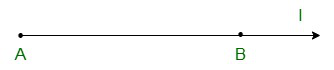### (ii) In how many points can two distinct lines at the most intersect?

Solution:

(i) An infinitely large number of lines can pass through a given point

(ii) Two distinct lines at the most intersect at one point.

### (ii) Name the line segments determined by the three collinear points P, Q and R.

Solution:

(i) One line segment is incident on the points P and Q.

(ii) PQ, QR, PR are line segments determined by the three collinear points P, Q and R.

Solution:

(i) False

(ii) False

(iii) False

(iv) True

(v) False

(vi) True

(vii) False

(viii) False

(ix) False

(x) False

### Question 5: In the below figure, name the following: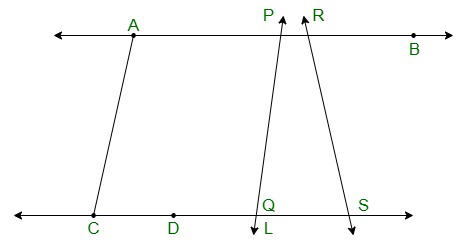### (iv) Two pairs of non–intersecting line segments

Solution:

(i) Five line segments, namely,  AB, CD, AC, PQ and DS respectively.

(ii) Five rays : PA, RB, DC, QS, DS

(iii) Four collinear points, namely, C, D, Q, S respectively.

(iv) Two pairs of non–intersecting line segments, which are AB and CD, PB and LS respectively.

### (iv) A line separates a plane into _________ parts namely the __________ and the _____ itself.

Solution:

(i) unique

(ii) lines

(iii) perpendicular, perpendicular

(iv) three, two half planes, line.

My Personal Notes arrow_drop_up